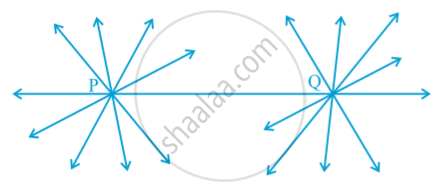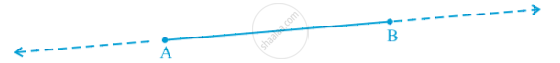# Euclid’S Definitions, Axioms and Postulates

#### notes

A solid has shape, size, position, and can be moved from one place to another. Its boundaries are called surfaces.

The three steps from solids to points (solids-surfaces-lines-points). In each step we lose one extension, also called a dimension.
He began his exposition by listing 23 definitions in Book 1 of the ‘Elements’. A few of them are given
below :
1. A point  is that which has no part.
2. A line is breadthless length.
3. The ends of a line are points.
4. A straight line is a line which lies evenly with the points on itself.
5. A surface is that which has length and breadth only.
6. The edges of a surface are lines.
7. A plane surface is a surface which lies evenly with the straight lines on itself.

Since it refers to breadth and length, neither of which has been defined. Because of this, a few terms are kept undefined while developing any course of study. So, in geometry, we take a point, a line and a plane (in Euclid‘s words a plane surface) as undefined terms. The only thing is that we can represent them intuitively, or explain them with the help of ‘physical models’.
Starting with his definitions, Euclid assumed certain properties, which were not to be proved. These assumptions are actually ‘obvious universal truths’. He divided them into two types: axioms and postulates. He used the term ‘postulate’ for the assumptions that were specific to geometry. Common notions (often called axioms), on the other hand, were assumptions used throughout mathematics and not specifically linked to geometry.
For details about axioms and postulates, Some of Euclid’s axioms, not in his order, are given below :
(1) Things which are equal to the same thing are equal to one another.
(2) If equals are added to equals, the wholes are equal.
(3) If equals are subtracted from equals, the remainders are equal.
(4) Things which coincide with one another are equal to one another.
(5) The whole is greater than the part.
(6) Things which are double of the same things are equal to one another.
(7) Things which are halves of the same things are equal to one another.
Now let us discuss Euclid’s five postulates. They are :
Postulate 1 : A straight line may be drawn from any one point to any other point.
Note that this postulate tells us that at least one straight line passes through two distinct points, but it does not say that there cannot be more than one such line. However, in his work, Euclid has frequently assumed, without mentioning, that there is a unique line joining two distinct points. We state this result in the form of an axiom as follows:

Axiom - Given two distinct points, there is a unique line that passes through them.in above fig. the lines passing through P also pass through Q is only one line  that is  PQ where as the lines passing through Q also pass through P is  only one line that is PQ.

Postulate 2 : A terminated line can be produced indefinitely.
Note that what we call a line segment now-a-days is what Euclid called a terminated line. So, according to the present day terms, the second postulate says that a line segment can be extended on either side to form a line in following fig.Postulate 3 : A circle can be drawn with any centre and any radius.
Postulate 4 : All right angles are equal to one another.
Postulate 5 : If a straight line falling on two straight lines makes the interior angles on the same side of it taken together less than two right angles, then the two straight lines, if produced indefinitely, meet on that side on which the sum of angles is less than two right angles.
A system of axioms is called consistent , if it is impossible to deduce from these axioms a statement that contradicts any axiom or previously proved statement. So, when any system of axioms is given, it needs to be ensured that the system is consistent.
After Euclid stated his postulates and axioms, he used them to prove other results. Then using these results, he proved some more results by applying deductive reasoning. The statements that were proved are called propositions or theorems.

If you would like to contribute notes or other learning material, please submit them using the button below.

### Shaalaa.com

Euclides axioms and postulates [00:22:16]
S
0%